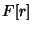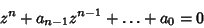## Number Field

Ifis an Algebraic Number of degree, then the totality of all expressions that can be constructed fromby repeated additions, subtractions, multiplications, and divisions is called a number field (or an Algebraic Number Field) generated by, and is denoted. Formally, a number field is a finite extensionof the Fieldof Rational Numbers.

The numbers of a number field which are Roots of a Polynomialwith integral coefficients and leading coefficient 1 are called the Algebraic Integers of that field.